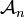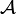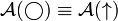# Notes for AKT-090924-1

Some dimensions of${\mathcal A}_n$,${\mathcal A}$ is a commutative algebra,${\mathcal A}(\bigcirc)\equiv{\mathcal A}(\uparrow)$.